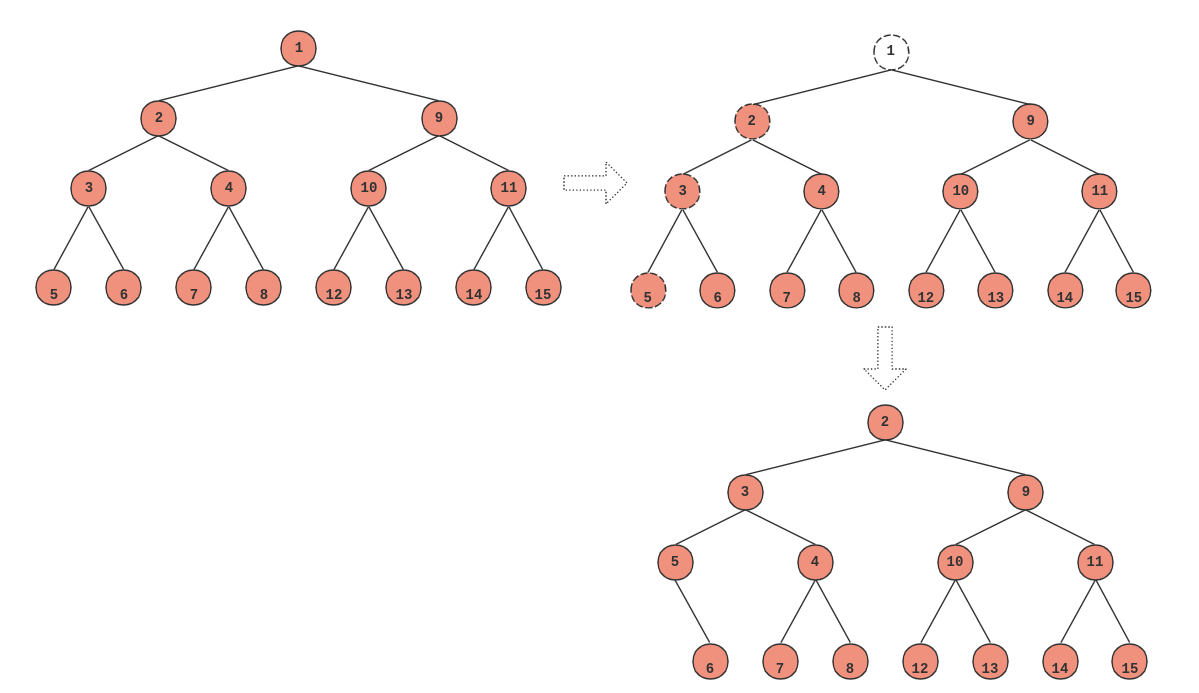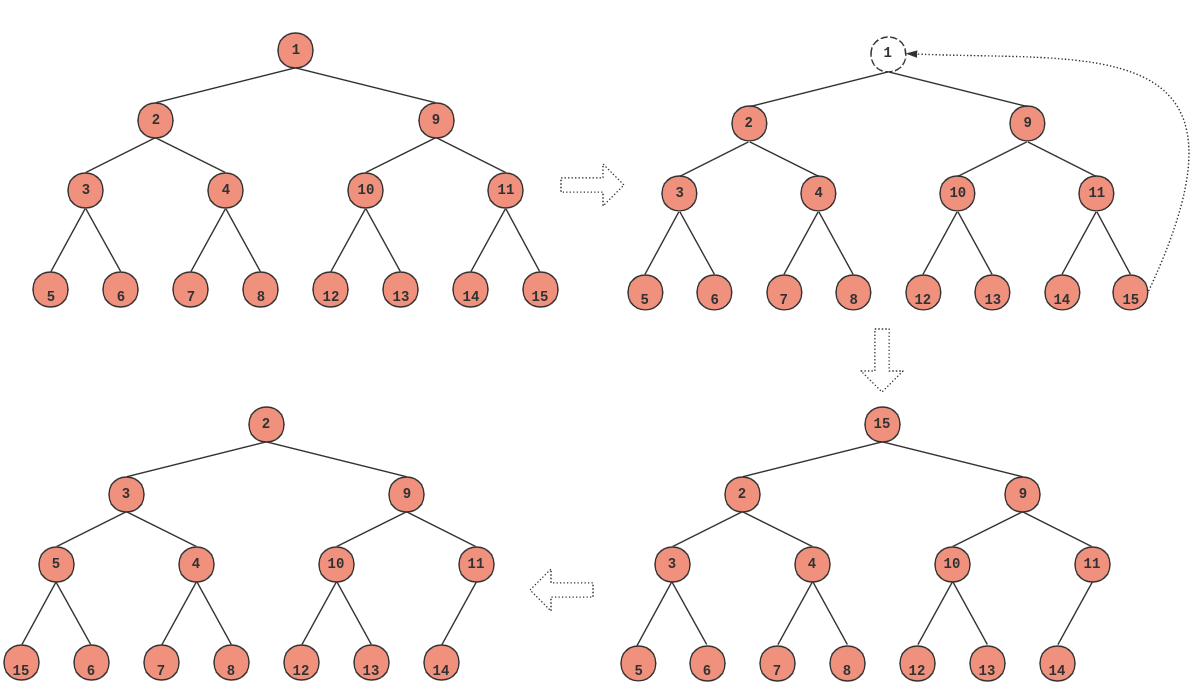## 1. heap 的使用

``[[email protected].io]# cat heap_sample00.go``heapObj := MinHeap{}``heap.Init(&heapObj)``heap.Push(&heapObj, &Item{Value: 1, Priority: 1})``item := heap.Pop().(*Item)``

• 创建 Heap 的底层数据存储结构，这里是创建了一个 Obj，其实内部是一个树组，等下会看到详情
• 调用了 heap 标准库的 `Init` 函数
• 通过 heap 的 Push 方法插入元素
• 通过 heap 的 Pop 方法取出堆顶元素

• 这个 Object 不是随意的，需要实现一个 Interface
• 必须通过 `heap.Init` 来初始化 Heap，这样，内部才是一个真正的堆结构
• 存取必须通过 Heap.Push 和 Pop 方法来操作

``[[email protected].io]# cat interface.go``type Interface interface {``    sort.Interface``    Push(x interface{}) // add x as element Len()``    Pop() interface{}   // remove and return element Len() - 1.``}``

sort.Interface 就很简单了，有三个函数需要实现，分别是：

``[[email protected].io]# cat sort.go``type Interface interface {``    Len() int``    Less(i, j int) bool``    Swap(i, j int)``}``

### Push 方法

Push 方法和 Pop 方法需要着重介绍一下，因为他们有一些隐藏的知识。

``[[email protected].io]# cat push.go``func (h *MaxHeap) Push(x interface{}) {``    *h = append(*h, x.(*Item))``}``

### Pop 方法

Pop 就有更多的规矩了，首先得保证 Pop 完之后，树组的元素是要少一个的，长度也要减掉，这个是由我们自己来实现的；然后，需要注意的是，heap 包已经帮我们把要移除的元素放在了最后，所以，只需要把最后一个元素返回去就可以了。

``[[email protected].io]# cat pop.h``func (h *MinHeap) Pop() interface{} {``    x := (*h)[len(*h)-1]``    *h = (*h)[0 : len(*h)-1]``    return x``}``

## 2. heap 的实现

### 2.1 init 函数

``[[email protected].io]# cat init.go``func Init(h Interface) {``    // heapify``    n := h.Len()``    for i := n/2 - 1; i >= 0; i-- {``        down(h, i, n)``    }``}``

### 2.2 down 函数

``[[email protected].io]# cat down.go``func down(h Interface, i0, n int) bool {``    i := i0``    for {``        j1 := 2*i + 1``        if j1 >= n || j1 < 0 { // j1 < 0 after int overflow``            break``        }``        j := j1 // left child``        if j2 := j1 + 1; j2 < n && h.Less(j2, j1) {``            j = j2 // = 2*i + 2  // right child``        }``        if !h.Less(j, i) {``            break``        }``        h.Swap(i, j)``        i = j``    }``    return i > i0``}``

i4 < i8
i2 < i4
i1 < i2
i0 < i1

i0 < i1 < i2 < i4 < i8

### 2.3 Push

``[[email protected].io]# cat push.go``// Push pushes the element x onto the heap.``// The complexity is O(log n) where n = h.Len().``func Push(h Interface, x interface{}) {``    h.Push(x)``    up(h, h.Len()-1)``}``

### 2.4 up

up 函数和 down 函数相对，down 是将一个元素不断得与自己子节点对比，从而实现父节点肯定比子节点小；而 up 函数则反过来，不断得将子节点与父节点对比，从而保证子节点比父节点大，代码为：

``[[email protected].io]# cat up.go``func up(h Interface, j int) {``    for {``        i := (j - 1) / 2 // parent``        if i == j || !h.Less(j, i) {``            break``        }``        h.Swap(i, j)``        j = i``    }``}``

### 2.5 Pop 函数## 3. 小结

• init 的时间复杂度最坏是 NlogN
• Pop 的操作时间复杂度是 logN

• Push 的操作时间复杂度也是 logN，最好是 O(1)。但是，在业界已经有人证明了，Push 的平均比较此书是 2.607 次，也就是说性能还不错。